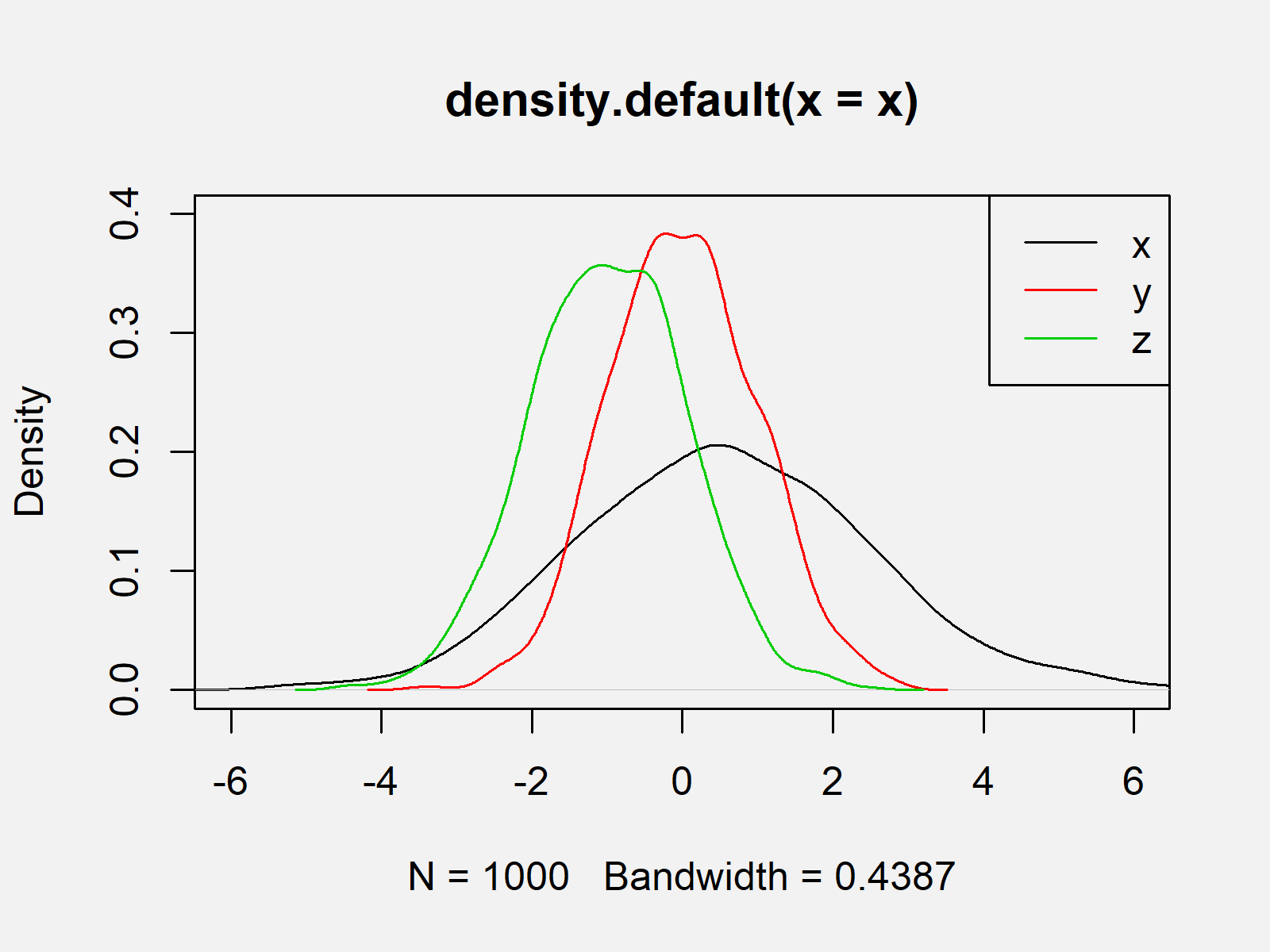# Overlay Density Plots in Base R (2 Examples)

In this article, I’ll show how to draw several Kernel densities in the same graphic in the R programming language.

The table of content is structured as follows:

So without further ado, let’s dive right in…

## Creation of Example Data

As a first step, let’s create some example data:

```set.seed(8723264)         # Create example data
x <- rnorm(1000, 0.5, 2)
y <- rnorm(1000)
z <- rnorm(1000, - 1)```

As you can see based on the previous RStudio console output, our example data contains of three randomly distributed numeric vectors.

## Example 1: Overlay Multiple Densities in R

This Example illustrates how to overlay multiple numeric distributions in the same graphic in the basic installation of the R programming language. For this task, we need to apply the plot, lines, and density functions as shown below. Note that the first density needs to be created using the plot function and all remaining densities need to be drawn with the lines function.

```plot(density(x),          # Plot density of x
xlim = c(- 6, 6),
ylim = c(0, 0.4))
lines(density(y),         # Add density of y
col = 2)
lines(density(z),         # Add density of z
col = 3)```The output of the previous R programming code is visualized in Figure 1: It shows the Kernel density plots of our three numeric vectors.

## Example 2: Add Legend to Plot with Multiple Densities

Based on Figure 1 you cannot know which of the lines correspond to which vector. Example 2 therefore illustrates how to add a legend to a graph showing multiple densities in R. If we want to draw a legend to a Base R plot, we can use the legend() function as follows:

```legend("topright",        # Add legend to plot
c("x", "y", "z"),
col = 1:3,
lty = 1)```As shown in Figure 2, we created the same plot again but this time showing a legend at the top right of our plot.

## Video & Further Resources

Would you like to know more about graphics in R? Then you could watch the following video of my YouTube channel. I’m explaining the examples of this tutorial in the video.

Furthermore, I can recommend to read the related tutorials on my homepage. Some tutorials can be found here:

In this article, I explained how to overlay multiple density plots in the R programming language. Let me know in the comments below, in case you have additional questions or comments.

Subscribe to the Statistics Globe Newsletter